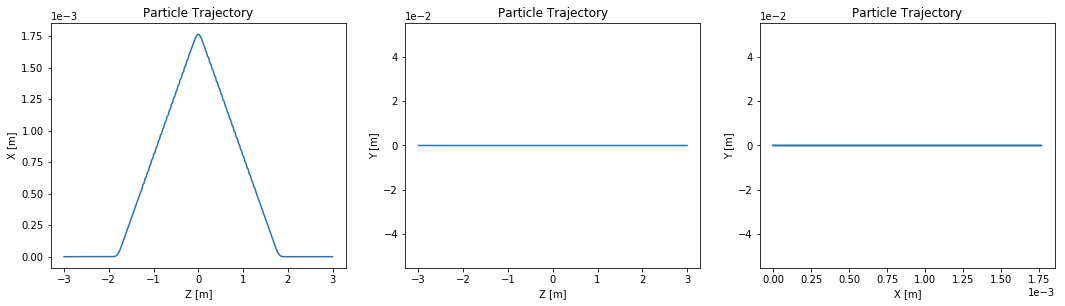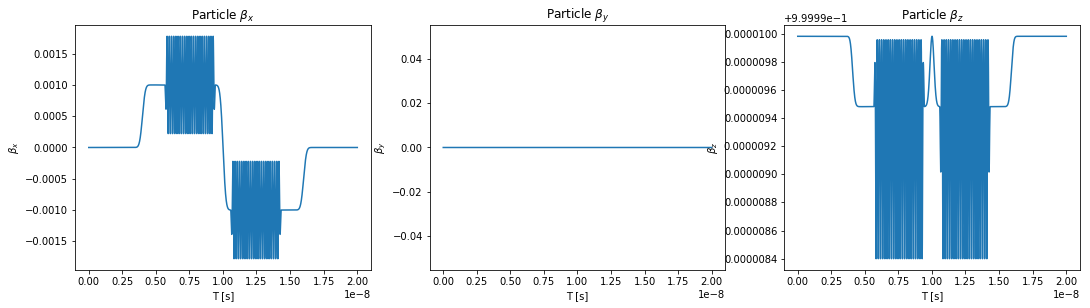# Example 005: Canted Striaght with 2 Undulators¶

In this example the electron trajectory in a mock-straight section with two undulators and center canting magnet is calculated in two ways. Firstly it is calculated with initial conditions given at z=0 (in the middle), then for the initial conditions given before the first undulator.

In :
# This has nothing to do with OSCARS, but it puts the matplotlib plots inline in the notebook
%matplotlib inline

# Import the OSCARS SR module
import oscars.sr

# Import basic plot utilities (matplotlib).  You don't need these to run OSCARS, but it's used here for basic plots
from oscars.plots_mpl import *

OSCARS v2.1.8 - Open Source Code for Advanced Radiation Simulation
Brookhaven National Laboratory, Upton NY, USA
http://oscars.bnl.gov
oscars@bnl.gov

In :
# Create a new OSCARS object.  Default to 8 threads and always use the GPU if available


## Create the undulator and Canting Magnet Fields¶

Here we create the undulator fields and Bending Magnet fields.

For the undulator, bfield represents maximum magnetic field [$B_x, B_y, B_z$]. The period is also in vector form which allows you to orient the axis of the undulator in any arbitrary direction. The number of periods is given by nperiods. This is the number of FULL periods. A terminating field of 1 period length is added to each side in addition to nperiods.

For the canting magnets we will use the gaussian field. bfield represents maximum magnetic field [$B_x, B_y, B_z$]. sigma is the 1-sigma width and can be given an any direction (if zero, that coordinate is ignored).

Typically clear_magnetic_fields() is called before adding a field in notebooks only to save time when making changes and rerunning sections of the code so it is not strictly necessary.

The undulators and canting magnets are placed in space using the translate option.

In :
# Clear any existing fields (just good habit in notebook style) and add an undulator field
osr.clear_bfields()
dist_between_centers = 0.049*15

osr.add_bfield_gaussian(bfield=[0, +0.08, 0], sigma=[0, 0, 0.05], translation=[0, 0, -1.8])
osr.add_bfield_undulator(bfield=[0, 1, 0], period=[0, 0, 0.049], nperiods=21, translation=[0, 0, -dist_between_centers])
osr.add_bfield_gaussian(bfield=[0, -0.16, 0], sigma=[0, 0, 0.05], translation=[0, 0, 0])
osr.add_bfield_undulator(bfield=[0, 1, 0], period=[0, 0, 0.049], nperiods=21, translation=[0, 0, +dist_between_centers])
osr.add_bfield_gaussian(bfield=[0, +0.08, 0], sigma=[0, 0, 0.05], translation=[0, 0, 1.8])

# Just to check the field that we added seems visually correct
plot_bfield(osr, -2, 2)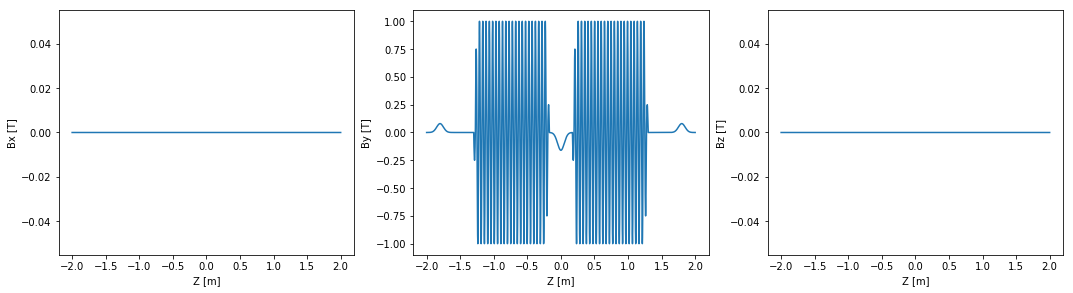Here we add a particle beam making use of some of the defaults, namely:

* type='electron'
* t0=0
* x0=[0, 0, 0]
* d0=[0, 0, 1]



For perfectly symmetric data sometimes it is convenient to set the initial conditions in the middle of your scenario. Often with real data it is more practical to set it before entry of the first device of interest. Both of these are shown below.

One must specify ctstartstop, and they are notebly different for the two examples of beam setups below.

clear_particle_beams() is called, again for convenience, but it is not necessary.

In :
# Setup beam similar to NSLSII
osr.clear_particle_beams()
osr.set_particle_beam(energy_GeV=3, current=0.500)

# Set the start and stop times for the calculation
osr.set_ctstartstop(-3, 3)


## Calculate Trajectory¶

Now we calculate the trajectory and plot it. It is enough to call calculate_trajectory(). If you are doing other calculations (flux, spectra, power density) it is not necesary to call this since it is called internally.

In :
# Run the particle trajectory calculation
trajectory = osr.calculate_trajectory()

# Plot the trajectory position and velocity
plot_trajectory_position(trajectory)
plot_trajectory_velocity(trajectory)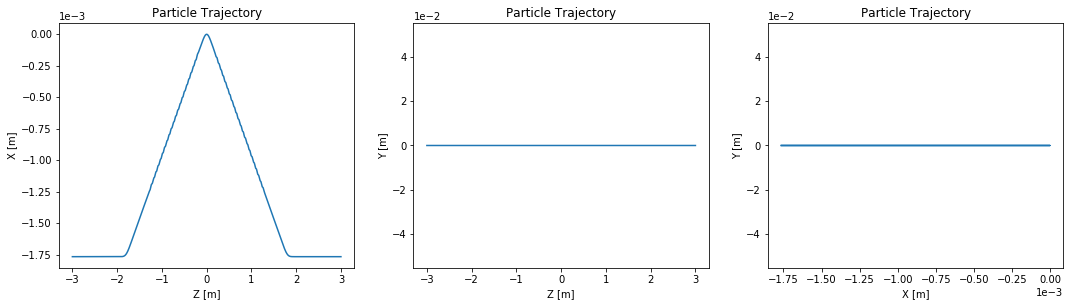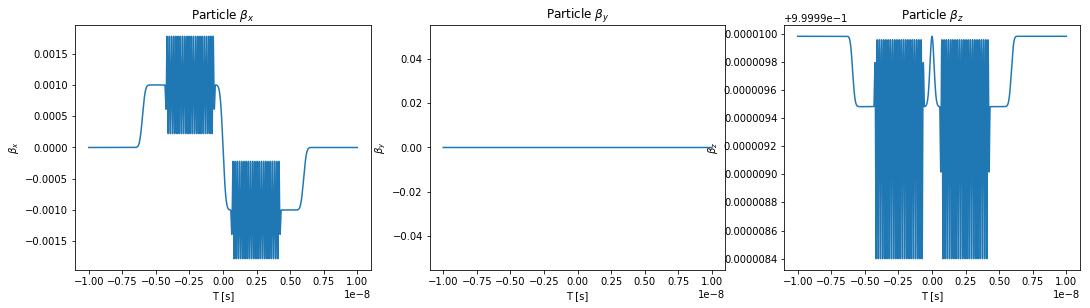Now set the initial conditions before entry of the first canting magnet (often this is more practical).

In :
# Setup beam similar to NSLSII with different starting position from above
# (this makes more sense for some scenarios)
osr.clear_particle_beams()
osr.set_particle_beam(x0=[0, 0, -3], energy_GeV=3, current=0.500)

# Set the start and stop times for the calculation
osr.set_ctstartstop(0, 6)

In :
# Run the particle trajectory calculation
trajectory = osr.calculate_trajectory()

# Plot the trajectory position and velocity
plot_trajectory_position(trajectory)
plot_trajectory_velocity(trajectory)